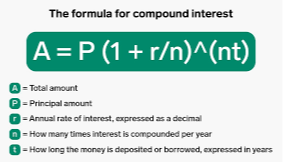# Compound Interest: Understanding the Power of Time and Money

May 17, 2023

Compound interest is a financial concept that plays a crucial role in the world of investments and savings. It refers to the interest earned not only on the principal amount but also on the accumulated interest over time. In simple terms, it is the interest on interest.

The concept of compound interest can be explained using a simple example. Suppose you invest \$1000 at an annual interest rate of 5%. At the end of the first year, you will earn \$50 in interest, making your total investment worth \$1050. In the second year, you will earn 5% interest on \$1050, which is \$52.50. At the end of the second year, your total investment will be worth \$1102.50. This cycle continues, and over time, the interest earned on the accumulated interest can significantly increase the value of your initial investment.

Compound interest is a powerful tool for investors because it can help them grow their wealth over time. However, it also works against those who borrow money because they end up paying more in interest than they initially borrowed. Therefore, it is essential to understand the concept of compound interest before making any financial decisions.

The formula for calculating compound interest is A = P(1 + r/n)^(nt), where A is the final amount, P is the principal amount, r is the annual interest rate, n is the number of times the interest is compounded per year, and t is the time period in years. This formula takes into account the effect of compounding on the final amount.

It is crucial to note that the frequency of compounding plays a significant role in the calculation of compound interest. The more frequently the interest is compounded, the higher the final amount will be. For example, if an investment earns 5% annual interest compounded monthly, it will earn more interest than an investment earning 5% annual interest compounded annually.

Another important factor to consider when dealing with compound interest is the concept of the time value of money. This concept states that the value of money decreases over time due to inflation. Therefore, the longer the time period, the lower the value of money. This means that the longer the investment period, the higher the interest rate required to compensate for inflation.

In conclusion, compound interest is a powerful financial tool that can help investors grow their wealth over time. However, it is essential to understand the concept and its implications before making any financial decisions. The frequency of compounding and the time value of money are crucial factors to consider when dealing with compound interest. By understanding these concepts, investors can make informed decisions and maximize their returns.  #invest #B1Financial #StuartBrisgel #fixedincome #preferedstock #bonds #treasury #modifiedduration #powerone